User:Tohline/SR/IdealGas

 |   Tiled Menu   |   Tables of Content   |  Banner Video   |  Tohline Home Page   |

Ideal Gas Equation of State

Much of the following overview of ideal gas relations is drawn from Chapter II of Chandrasekhar's classic text on Stellar Structure [C67], which was originally published in 1939. A guide to parallel print media discussions of this topic is provided alongside the ideal gas equation of state in the key equations appendix of this H_Book.

Fundamental Properties of an Ideal Gas

Property #1

An ideal gas containing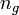$~n_g$ free particles per unit volume will exert on its surroundings an isotropic pressure (i.e., a force per unit area)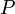$~P$ given by the following

Standard Form
of the Ideal Gas Equation of State,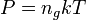$~P = n_g k T$

[C67], Chapter VII.3, Eq. (18)
D. D. Clayton (1968), Eq. (2-7)
[H87], §1.1, p. 5

if the gas is in thermal equilibrium at a temperature$~T$.

Property #2

The internal energy per unit mass$~\epsilon$ of an ideal gas is a function only of the gas temperature$~T$, that is,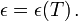$~\epsilon = \epsilon(T) \, .$

[C67], Chapter II, Eq. (1)

Specific Heats

Drawing from Chapter II, §1 of [C67]:  "Let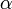$~\alpha$ be a function of the physical variables. Then the specific heat,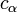$~c_\alpha$, at constant$~\alpha$ is defined by the expression,"$~c_\alpha$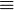$~\equiv$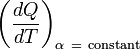$~\biggl( \frac{dQ}{dT} \biggr)_{\alpha ~=~ \mathrm{constant}}$

The specific heat at constant pressure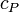$~c_P$ and the specific heat at constant (specific) volume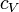$~c_V$ prove to be particularly interesting parameters because they identify experimentally measurable properties of a gas.

From the Fundamental Law of Thermodynamics, namely,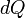$~dQ$$~=$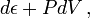$~ d\epsilon + PdV \, ,$

it is clear that when the state of a gas undergoes a change at constant (specific) volume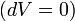$~(dV = 0)$,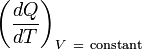$~\biggl( \frac{dQ}{dT} \biggr)_{V ~=~ \mathrm{constant}}$$~=$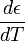$~\frac{d\epsilon}{dT}$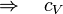$~\Rightarrow ~~~ c_V$$~=$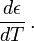$~\frac{d\epsilon}{dT} \, .$

Assuming$~c_V$ is independent of$~T$ — a consequence of the kinetic theory of gasses; see, for example, Chapter X of [C67] — and knowing that the specific internal energy is only a function of the gas temperature — see Property #2 above — we deduce that,$~\epsilon$$~=$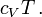$~c_V T \, .$

[C67], Chapter II, Eq. (10)
[LL75], Chapter IX, §80, Eq. (80.10)
[H87], §1.2, p. 9
[HK94], §3.7.1, immediately following Eq. (3.80)

Also, from Form A of the Ideal Gas Equation of State (see below) and the recognition that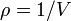$~\rho = 1/V$, we can write,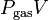$~P_\mathrm{gas}V$$~=$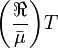$~\biggl(\frac{\Re}{\bar\mu} \biggr) T$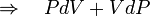$~\Rightarrow ~~~ PdV + VdP$$~=$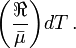$~\biggl(\frac{\Re}{\bar\mu} \biggr) dT \, .$

As a result, the Fundamental Law of Thermodynamics can be rewritten as,$~dQ$$~=$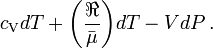$~c_\mathrm{V} dT + \biggl(\frac{\Re}{\bar\mu} \biggr) dT - VdP \, .$

This means that the specific heat at constant pressure is given by the relation,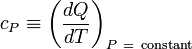$~c_P \equiv \biggl( \frac{dQ}{dT} \biggr)_{P ~=~ \mathrm{constant}}$$~=$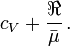$~c_V + \frac{\Re}{\bar\mu} \, .$

That is,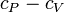$~c_P - c_V$$~=$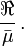$~\frac{\Re}{\bar\mu} \, .$

[C67], Chapter II, §1, Eq. (9)
D. D. Clayton (1968), Eq. (2-108)
[LL75], Chapter IX, §80, immediately following Eq. (80.11)
[H87], §1.2, p. 9
[KW94], §4.1, immediately following Eq. (4.15)

Consequential Ideal Gas Relations

Throughout most of this H_Book, we will define the relative degree of compression of a gas in terms of its mass density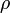$~\rho$ rather than in terms of its number density$~n_g$. Following D. D. Clayton (1968) — see his p. 82 discussion of The Perfect Monatomic Nondegenerate Gas — we will "let the mean molecular weight of the perfect gas be designated by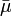$~\bar{\mu}$. Then the density is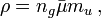$~\rho = n_g \bar\mu m_u \, ,$

where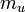$~m_u$ is the mass of 1 amu" (atomic mass unit). "The number of particles per unit volume can then be expressed in terms of the density and the mean molecular weight as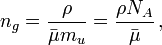$~n_g = \frac{\rho}{\bar\mu m_u} = \frac{\rho N_A}{\bar\mu} \, ,$

where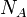$~N_A$ = 1/$~m_u$ is Avogadro's number …" Substitution into the above-defined Standard Form of the Ideal Gas Equation of State gives, what we will refer to as,

Form A
of the Ideal Gas Equation of State,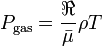$~P_\mathrm{gas} = \frac{\Re}{\bar{\mu}} \rho T$

[LL75], Chapter IX, §80, Eq. (80.8)
[KW94], §2.2, Eq. (2.7) and §13, Eq. (13.1)

where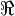$~\Re$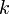$~k$$~N_A$ is generally referred to in the astrophysics literature as the gas constant. The definition of the gas constant can be found in the Variables Appendix of this H_Book; its numerical value can be obtained by simply scrolling the computer mouse over its symbol in the text of this paragraph. See §VII.3 (p. 254) of [C67] or §13.1 (p. 102) of [KW94] for particularly clear explanations of how to calculate$~\bar{\mu}$.

Employing a couple of the expressions from the above discussion of specific heats, the right-hand side of Form A of the Ideal Gas Equation of State can be rewritten as,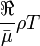$~\frac{\Re}{\bar\mu} \rho T$$~=$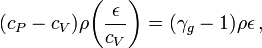$~ (c_P - c_V)\rho \biggl(\frac{\epsilon}{c_V}\biggr) = (\gamma_g - 1)\rho\epsilon \, ,$

where we have — as have many before us — introduced a key physical parameter,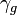$~\gamma_g$$~\equiv$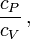$~\frac{c_P}{c_V} \, ,$

[C67], Chapter II, immediately following Eq. (9)
[LL75], Chapter IX, §80, immediately following Eq. (80.9)
[T78], §3.4, immediately following Eq. (72)
[HK94], §3.7.1, Eq. (3.86)

to quantify the ratio of specific heats. This leads to what we will refer to as,

Form B
of the Ideal Gas Equation of State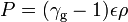$~P = (\gamma_\mathrm{g} - 1)\epsilon \rho$

[C67], Chapter II, Eq. (5)
[HK94], §1.3.1, Eq. (1.22)
[BLRY07], §6.1.1, Eq. (6.4)

Related Wikipedia Discussions

 © 2014 - 2019 by Joel E. Tohline |   H_Book Home   |   YouTube   | Context: | PGE | SR | Appendices: | Equations | Variables | References | Binary Polytropes | Ramblings | Images | Images (2016 Layout) | ADS |